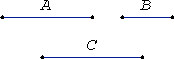# Proposition 10

Of magnitudes which have a ratio to the same, that which has a greater ratio is greater; and that to which the same has a greater ratio is less.

Let A have to C a greater ratio than B has to C.

I say that A is greater than B.

If not, then A either equals B or is less than it.V.7

Now A does not equal B, for in that case each of the magnitudes A and B would have the same ratio to C, but they do not, therefore A does not equal B.

V.8

Nor is A less than B, for in that case A would have to C a less ratio than B has to C, but it does not, therefore A is not less than B.

But it was proved not to be equal either, therefore A is greater than B.

Next, let C have to B a greater ratio than C has to A.

I say that B is less than A.

If not, it is either equal or greater.

V.7

Now B does not equal A, for in that case C would have the same ratio to each of the magnitudes A and B, but it does not, therefore A does not equal B.

V.8

Nor is B greater than A, for in that case C would have to B a less ratio than it has to A, but it does not, therefore B is not greater than A.

But it was proved that it is not equal either, therefore B is less than A.

Therefore, of magnitudes which have a ratio to the same, that which has a greater ratio is greater; and that to which the same has a greater ratio is less.

Q.E.D.

## Guide

This converse to proposition V.8 has two statements.

If a : c > b : c, then a > b.
If c : b > c : a, then b < a.

Part of the law of trichotomy for ratios is used in this proof, the part which says at most one of the three cases a : c < b : c, a : c = b : c, or a : c > b : c, can occur.

Euclid’s proof relies on using V.Def.4 as an axiom of comparability since it uses proposition V.8 and the law of trichotomy for ratios. But the proposition can also be proved without the axiom.

Suppose a : c > b : c. Then there are numbers m and n such that na > mc but nb is not greater than mc. Therefore na > nb. Therefore a > b. Thus a : c > b : c implies a > b.
The other implication of the proposition can be proved similarly.

This proposition is used a few times in book V starting with V.14.